# Solutions, mixtures

We have 2 liters of 20% solution available. How much 70% solution do we need to add to it to get a 30% solution?

x =  0.5 l

### Step-by-step explanation:

2•0.20 + 0.70 • x = 0.30(2+x)

2•0.20 + 0.70 • x = 0.30•(2+x)

0.4x = 0.2

x = 1/2 = 0.5

Our simple equation calculator calculates it.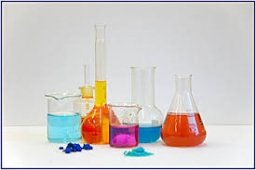Did you find an error or inaccuracy? Feel free to write us. Thank you!Tips to related online calculators
Do you have a linear equation or system of equations and looking for its solution? Or do you have a quadratic equation?
Tip: Our volume units converter will help you with the conversion of volume units.

## Related math problems and questions:

• Solutions, mixturesHow many liters of 70% solution we must add to 5 liters of 30% solution to give us a 60% solution?
• GramsHow many grams of 5% solution do we need to add to 100g of 50% solution to get a 20% solution?
• FertilizerI have 4 liters of 10% fertilizer. How much water do I need to add to have a 2% solution?
• Clogging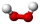How much-distilled water must the pharmacist add to 30g of a 30% hydrogen peroxide solution to obtain a 3% solution to clogging?
• Pouring alcohol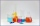100 liters of alcohol has 70% How many liters of water need to be added to have 60% alcohol?
• Mixture 2How many liters of water must be added to 7 liters of a 20% solution to obtain a 10% solution?
• Alcohol solutionsWe have to produce 2 liters of 60% alcohol from 55% and 80%. How many of which ones will we use in the solution?
• Copper sulphate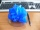How much g of water do we have to add to 240 g of an 84% CuSO4 solution to produce a 60% solution? (Express the mass of crystalline CuSO4 in the original solution and in the resulting solution and compare them. )
• SolutionsHow much 60% solution and how much 35% solution is needed to create 100 l of 40% solution?
• PeroxideHow many ml should we pour 30% of peroxide (H2O2) into 100ml H2O to give a 20% solution?
• Alcohol 2Two types of alcohol one 63% and second 75% give 20 liters of 69% alcohol. How many liters of each type are in the mixture?
• AlcoholHow many 55% alcohol we need to pour into 14 liters 75% alcohol to get p3% alcohol? How many 65% alcohol we get?
• CuSO4 mixtureHow many grams of solid CuSO4 we have to add to 450g of 15% CuSO4 solution to produce a 25% solution?
• Water solutionHow many grams of salt should we dissolve in 400 g of water to get a 20% solution?
• Fertilizer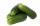Mr. Gherkin use for fertilization 7% solution of fertilizer. 17 liters of it still left. How much water must be added to the solution to make only 3% solution?
• Disinfecting solutionHow much distilled water is necessary to pour into 500 ml of 33% hydrogen peroxide solution to obtain 3% disinfecting solution?
• Milk mixing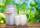You have 12 liters of milk with a temperature of 20°C. How much milk at 80°C do you have to add to have milk at 30°C?